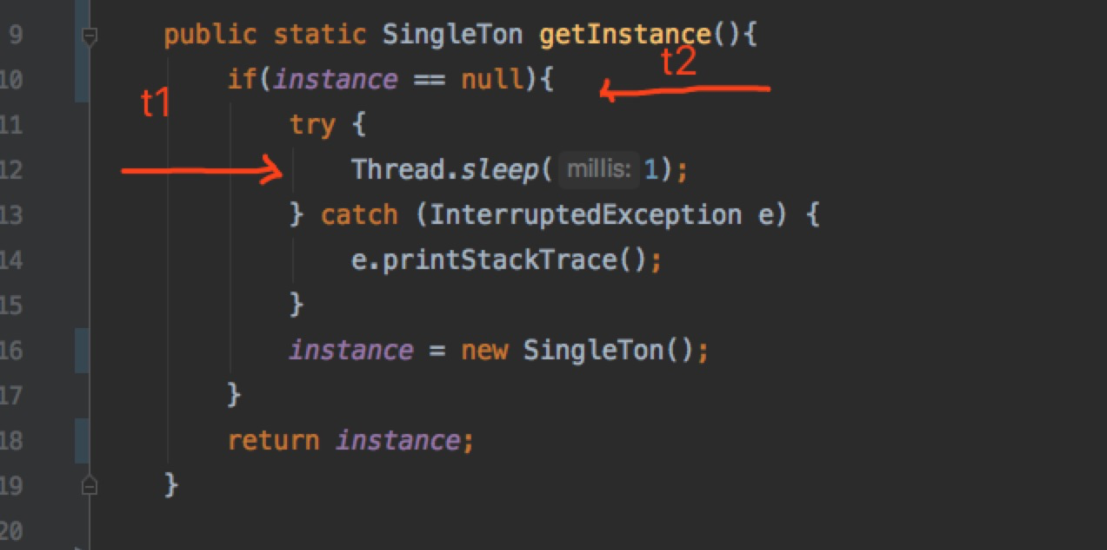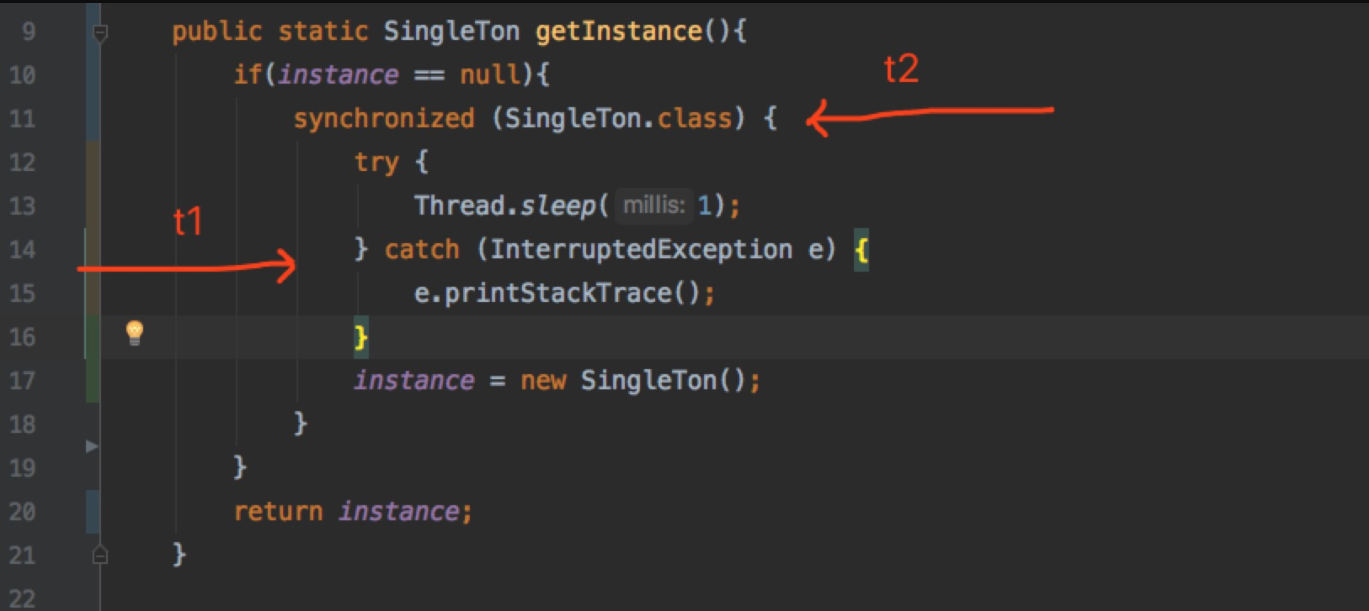### 8种单例模式写法助你搞定面试

• 面试宝典
• 时间：2020-10-31 17:53
• 319人已阅读

#### ：1. 单例模式常见问题

1.单例类如何控制其实例化

2.如何确保只有一个实例

private构造函数,类的实例话不对外开放,由自己内部来完成这个操作,确保永远不会从类外部实例化类,避免外部随意new出来新的实例。

#### 3. 单例模式8种写法

1.在多线程环境下行为是否线程安全

2.饿汉以及懒汉

3.编码是否优雅(理解起来是否比较直观)

1. 饿汉式线程安全的

public class SingleTon{

//私有 static final的

private static final SingleTon INSTANCE = new SingleTon();

//私有构造器

private SingleTon(){ }

public static SingleTon getInstance(){

return INSTANCE; }

public static void main(String[] args) {        SingleTon instance1 = SingleTon.getInstance();        SingleTon instance2 = SingleTon.getInstance();        System.out.println(instance1 == instance2);    } }

2. 饿汉式线程安全(变种写法)

public class SingleTon{

private static final SingleTon INSTANCE ;

static {    INSTANCE = new SingleTon(); }

private SingleTon(){}

public static SingleTon getInstance(){

return INSTANCE; }

public static void main(String[] args) {        SingleTon instance1 = SingleTon.getInstance();        SingleTon instance2 = SingleTon.getInstance();        System.out.println(instance1 == instance2);    } }

3. 懒汉式线程不安全

public class SingleTon{

private static  SingleTon instance ;

private SingleTon(){}

public static SingleTon getInstance(){

if(instance == null){                instance = new SingleTon();            }

return instance; }

public static void main(String[] args) {        SingleTon instance1 = SingleTon.getInstance();        SingleTon instance2 = SingleTon.getInstance();        System.out.println(instance1 == instance2);                // 通过开启100个线程 比较是否是相同对象        for(int i=0;i<100;i++){             new Thread(()->                System.out.println(SingleTon.getInstance().hashCode())            ).start();        }            } }

// 通过开启100个线程 比较是否是相同对象

for(int i=0;i<100;i++){

new Thread(()->                System.out.println(SingleTon.getInstance().hashCode())            ).start();        }

public static SingleTon getInstance(){

if(instance == null){

try {               Thread.sleep(1);           } catch (InterruptedException e) {               e.printStackTrace();           }           instance = new SingleTon();       }

return instance;   }4. 懒汉式线程安全(粗粒度Synchronized)

```public class SingleTon{

private static  SingleTon instance ;

private SingleTon(){}

public static SingleTon synchronized getInstance(){
if(instance == null){
instance = new SingleTon();
}
return instance;
}

public static void main(String[] args) {
SingleTon instance1 = SingleTon.getInstance();
SingleTon instance2 = SingleTon.getInstance();
System.out.println(instance1 == instance2);
// 通过开启100个线程 比较是否是相同对象
for(int i=0;i<100;i++){
new Thread(()->
System.out.println(SingleTon.getInstance().hashCode())
).start();
}

}

}```

5. 懒汉式线程不安全(synchronized代码块)

public class SingleTon{

private static  SingleTon instance ;

private SingleTon(){}

public static SingleTon getInstance(){

if(insatnce == null){

//这里锁住的是类class对象

synchronied(SingleTon.class){                    instance = new SingleTon();        }    }

return instance; }

public static void main(String[] args) {        SingleTon instance1 = SingleTon.getInstance();        SingleTon instance2 = SingleTon.getInstance();        System.out.println(instance1 == instance2);                // 通过开启100个线程 比较是否是相同对象        for(int i=0;i<100;i++){             new Thread(()->                System.out.println(SingleTon.getInstance().hashCode())            ).start();        }            } }6. 懒汉式线程安全(双重检验加锁)

public class SingleTon{

private static  volatile SingleTon instance ;

private SingleTon(){}

public static SingleTon getInstance(){

if(instance == null){

synchronied(SingleTon.class){

//这里在校验一次，就是懒汉式的

if(instance == null){                        instance = new SingleTon();                    }        }    }

return instance; }

public static void main(String[] args) {        SingleTon instance1 = SingleTon.getInstance();        SingleTon instance2 = SingleTon.getInstance();        System.out.println(instance1 == instance2);                // 通过开启100个线程 比较是否是相同对象        for(int i=0;i<100;i++){             new Thread(()->                System.out.println(SingleTon.getInstance().hashCode())            ).start();        }            } }

• 第一个判空(外层)的作用 ？

• 第二个判空(内层)的作用 ？

• 为什么变量修饰为volatile ？

1.分配内存

2.初始化构造器

3.将对象指向分配的内存的地址

7. 静态内部类的方式(基本完美了)

public class SingleTon{

public static SingleTon getInstance(){

return StaticSingleTon.instance;     }

private static class StaticSingleTon{

private static final SingleTon instance = new SingleTon();     }

public static void main(String[] args) {        SingleTon instance1 = SingleTon.getInstance();        SingleTon instance2 = SingleTon.getInstance();        System.out.println(instance1 == instance2);                // 通过开启100个线程 比较是否是相同对象        for(int i=0;i<100;i++){             new Thread(()->                System.out.println(SingleTon.getInstance().hashCode())            ).start();        }            } }

• 因为一个类的静态属性只会在第一次加载类时初始化，这是JVM帮我们保证的，所以我们无需担心并发访问的问题。所以在初始化进行一半的时候，别的线程是无法使用的，因为JVM会帮我们强行同步这个过程。

• 另外由于静态变量只初始化一次，所以singleton仍然是单例的。

8. 枚举类型的单例模式(太完美以至于。。。)

public Enum SingleTon{    INSTANCE;

public static void main(String[] args) {

// 通过开启100个线程 比较是否是相同对象        for(int i=0;i<100;i++){

new Thread(()->                System.out.println(SingleTon.getInstance().hashCode())            ).start();        }            } }

https://www.cnblogs.com/wyc1994666/p/11394755.html

Top Top